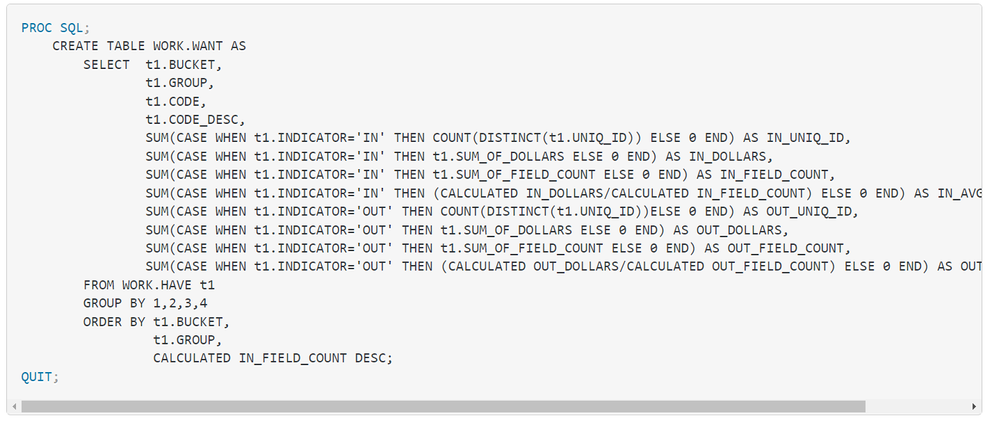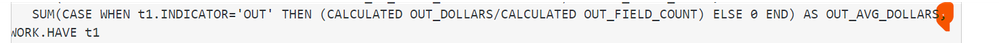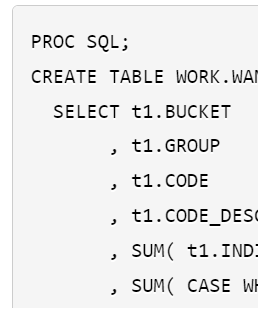## Count Distinct nested within Sum Function based on Case When Statement

Hi,

I coded the following thinking it would work but unfortunately SAS apparently cannot do what I am trying to do. Is there another way to accomplish this table?

``````PROC SQL;
CREATE TABLE WORK.WANT AS
SELECT  t1.BUCKET,
t1.GROUP,
t1.CODE,
t1.CODE_DESC,
SUM(CASE WHEN t1.INDICATOR='IN' THEN COUNT(DISTINCT(t1.UNIQ_ID)) ELSE 0 END) AS IN_UNIQ_ID,
SUM(CASE WHEN t1.INDICATOR='IN' THEN t1.SUM_OF_DOLLARS ELSE 0 END) AS IN_DOLLARS,
SUM(CASE WHEN t1.INDICATOR='IN' THEN t1.SUM_OF_FIELD_COUNT ELSE 0 END) AS IN_FIELD_COUNT,
SUM(CASE WHEN t1.INDICATOR='IN' THEN (CALCULATED IN_DOLLARS/CALCULATED IN_FIELD_COUNT) ELSE 0 END) AS IN_AVG_DOLLARS,
SUM(CASE WHEN t1.INDICATOR='OUT' THEN COUNT(DISTINCT(t1.UNIQ_ID))ELSE 0 END) AS OUT_UNIQ_ID,
SUM(CASE WHEN t1.INDICATOR='OUT' THEN t1.SUM_OF_DOLLARS ELSE 0 END) AS OUT_DOLLARS,
SUM(CASE WHEN t1.INDICATOR='OUT' THEN t1.SUM_OF_FIELD_COUNT ELSE 0 END) AS OUT_FIELD_COUNT,
SUM(CASE WHEN t1.INDICATOR='OUT' THEN (CALCULATED OUT_DOLLARS/CALCULATED OUT_FIELD_COUNT) ELSE 0 END) AS OUT_AVG_DOLLARS,
FROM WORK.HAVE t1
GROUP BY 1,2,3,4
ORDER BY t1.BUCKET,
t1.GROUP,
CALCULATED IN_FIELD_COUNT DESC;
QUIT;``````

I get the following errors:

``````ERROR: Result of WHEN clause 2 is not the same data type as the preceding results.
ERROR: Summary functions nested in this way are not supported.``````

Any help here is much appreciated! Thank you in advance!

2 REPLIES 2

## Re: Count Distinct nested within Sum Function based on Case When Statement

Hi @mhoward2,

To avoid the nesting of the summary functions SUM() and COUNT(DISTINCT ...) I suggest:

``COUNT(DISTINCT CASE WHEN t1.INDICATOR='IN' THEN t1.UNIQ_ID ELSE ' ' END) AS IN_UNIQ_ID``

(and analogously for 'OUT').

Regarding the error message about inconsistent data types, make sure that the THEN and ELSE branch result in the same data type. The code above assumes that UNIQ_ID is a character variable, hence the character missing in the ELSE branch. If UNIQ_ID is numeric, then use ELSE . END.

To avoid the nesting of the SUM() functions (via a CALCULATED item) I suggest:

``CALCULATED IN_DOLLARS/CALCULATED IN_FIELD_COUNT AS IN_AVG_DOLLARS``

(and analogously for 'OUT'). Please check if the results match your intentions.

Also remove the comma after the last SELECT item to avoid a syntax error.

## Re: Count Distinct nested within Sum Function based on Case When Statement

Does the source data have multiple observations per UNIQ_ID * INDICATION combination?

If not then the problem a bit easier.

``````PROC SQL;
CREATE TABLE WORK.WANT AS
SELECT t1.BUCKET
, t1.GROUP
, t1.CODE
, t1.CODE_DESC
, SUM( t1.INDICATOR='IN' ) as IN_UNIQ_ID
, SUM( CASE WHEN t1.INDICATOR='IN' THEN t1.SUM_OF_DOLLARS ELSE . END) AS IN_DOLLARS
, MEAN( CASE WHEN t1.INDICATOR='IN' THEN t1.SUM_OF_DOLLARS ELSE . END) AS IN_AVG_DOLLARS
, SUM( t1.INDICATOR='OUT' ) as OUT_UNIQ_ID
, SUM( CASE WHEN t1.INDICATOR='OUT' THEN t1.SUM_OF_DOLLARS ELSE . END) AS OUT_DOLLARS
, MEAN( CASE WHEN t1.INDICATOR='OUT' THEN t1.SUM_OF_DOLLARS ELSE . END) AS OUT_AVG_DOLLARS
FROM HAVE t1
GROUP BY BUCKET
, GROUP
, IN_FIELD_COUNT DESC
;
QUIT;
``````

PS:   Hiding separators at the end of the line, like in your original posting:Make it really difficult for humans to notice extra (or missing) separators:It is much easier to just scan down the evenly positioned left side of the lines to check for the separators:Discussion stats
• 2 replies
• 294 views
• 0 likes
• 3 in conversation